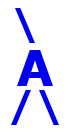## AIRES library reference: ghfin.

```c
c     Routine directly callable from C/C++ (it could be necessary to
c     append an underscore, "_", to its name depending on the C/C++
c     compiler used).
c
c
function ghfin(n, prepost)
c
c     Evaluating the inverse of the Gaisser-Hillas function (T. K.
c     Gaisser and A. M. Hillas, in Proc. 15th ICRC (Plovdiv), vol. 8,
c     p. 353 (1977))., that is, X such that ghf(X) = n.
c     The four parameters Nmax, Xmax, X0 and lambda are set by means of
c     the routine "ghfpars".
c
c     Written by: S. J. Sciutto, La Plata 2000.
c
c
c     Arguments:
c     =========
c
c     n............... (input, double precision) The number of
c                      particles. If n < 0 or n > Nmax, the
c                      result is a large negative number.
c     prepost......... (input, integer) Integer parameter labelling
c                      which of the two abscissas X has to be
c                      returned: If prepost <= 0 then X < Xmax;
c                      otherwise x > Xmax.
c                      Notice that the inverse of the gh function
c                      is bi-valuated.
c
c
c     Return value: (double precision) The value of the inverse
c     ============  gh function (in g/cm2), that is, X such that
c                   ghf(X) = n.
c
c
c<--->
```

 These pages are maintained by Sergio J. Sciutto Back to AIRES library index Back to AIRES home